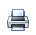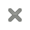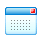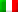# Courses

Course: :
Teacher:

### SPECIAL   ▾  N : 4

Course nameNotePeriodTime
Table
ISTITUZIONI DI DIDATTICA, LOGICA E STORIA DELLA MATEMATICA#### Istituzioni di Didattica, Logica e Storia della Matematica

Tema 1. Didattica: ( docente benedetto Scoppola , sem II)
- algoritmo di euclide in aritmetica
(massimo comun divisore) e nella teoria
dei numeri (frazioni continue)
- proporzioni ad elementi interi: i risultati
del libro VII
- potenze e proporzioni continue
- incommensurabili.
Tema 2. Logica:( docente Lorenzo Tortora de Falco, sem II)
- Soddisfacibilità e dimostrabilità. Il
teorema fondamentale dell'analisi
canonica.
- Gentzen e l'eliminazione del taglio
- Dimostrazioni e programmi: la
corrispondenza di Curry-Howard
SI
- Introduzione alla Logica Lineare.
Tema 3. Storia:( docente Enrico Rogora, sem I)
- Lagrange
- Il teorema di Ruffini Abel
- Il lavoro di Abel sulle equazioni e sulle
funzioni ellittiche
- Il contributo di Galois
- Le soluzioni analitiche delle equazioni di
- La teoria di Galois-Klein

• Benedetto Scoppola ( - Universita di Tor Vergata )
• Lorenzo TORTORA DE FALCO ( lorenzo.tortoradefalco@uniroma3.it - Dipartimento di Matematica e Fisica )
• Rogora Enrico ( enrico.rogora@uniroma1.it - Universita La Sapienza )
TIME SERIES ANALYSIS#### Time series analysis

1. We will recall the basic principles of applied and numerical Fourier analysis: Fourier series and transform, energy and power spectrum, mutual and autocorrelation and their numerical computation.
2. Impulse and harmonic response of a system.
3. Filtering of a time series.
4. Time series as sampling of a continuous signal.

• Roberto FERRETTI ( roberto.ferretti@uniroma3.it - Dipartimento di Matematica e Fisica )
GEOMETRY AND MECHANICS#### Geometry and Mechanics

The goal of these lessons is to show the relashionships between Continuum Physics and Differential Geometry, starting from the fundamentals of Mechanics. In particular, we show how any typical theory of mathematical-physics is based on two layers: the physical layer – the phenomenon under investigation, and the mathematical model used to represent the physics. We shall discuss in detail some model problems, starting from the theoretical point of view, up to some noteworthy solutions; for each model, we shall show the dual role, mathematical and physical, of the notions that are used. Model problems will be selected together with the students, and chosen among:
·       Active Soft Matter;
·       Liquid Crystals;
·       Solid - Fluid interactions.

Synopsis
- A continuum body as a differentiable manifold.
- Geometric elements; change of densities.-
- Geometric meaning of Divergence and Gradient
- Tell the difference between tensors: strain tensor versus stress tensor.
- Pull back & push forward of scalar, vector and tensor fields.
- Principle of virtual power:  the notion of force as a power gauge.
- Power versus Energy.
- Dissipation principle.
- Frame invariance.

semester II

• Luciano TERESI ( luciano.teresi@uniroma3.it - Dipartimento di Matematica e Fisica )

Nov, Dec, 2023• Vincenzo BONIFACI ( vincenzo.bonifaci@uniroma3.it - Dipartimento di Matematica e Fisica )

Questa pagina è disponibile in:Italiano